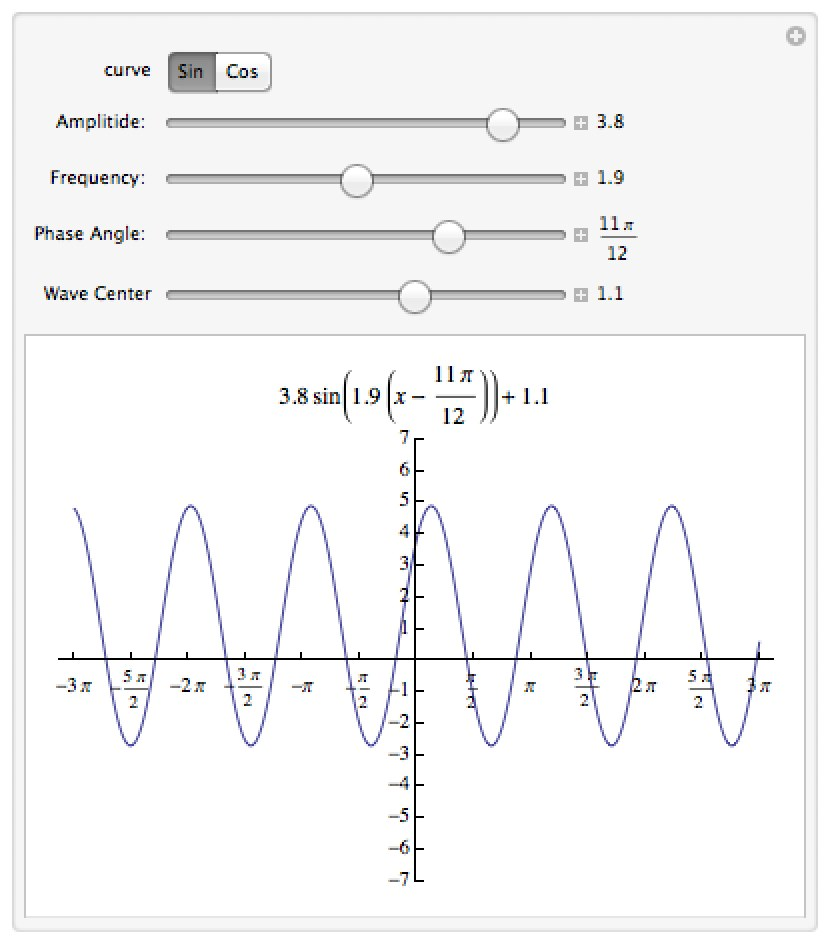# Mathematica Notebooks for Trigonometry

Important Note: The links for the notebooks open a new window or tab with a Google Drive page – the current settings for our homepages won’t allow me to host mathematica notebooks locally.

## Trigonometric Waves

This notebook lets you play with the amplitude, frequency, phase angle, and vertical shift of a sinusoidal wave and see what that does to its equation.## Exact values for Sine and Cosine

This notebook lets you see the exact values for sine and cosine at an integer number of degrees.  The slider lets you find the values for angles whose measure is a multiple of 3; opening the slider control and entering any integer will let you see the values for other angles (like sin(1°)).  A version of this can be found in the Wolfram Demonstrations Project.